## 图像处理之高斯滤波

2021/1/28 23:31:35 文章标签:

1. 高斯函数与高斯滤波 一维高斯函数我们都熟悉&#xff0c;形式如下&#xff1a; G(x)12πσexp⁡(−x22σ2)G(x) \frac{1}{\sqrt{2\pi}\sigma} \exp(-\frac{x^2}{2\sigma^2})G(x)2π​σ1​exp(−2σ2x2​) 计算机视觉中&#xff0c;高斯滤波使用的高斯核为x和y两个一维高…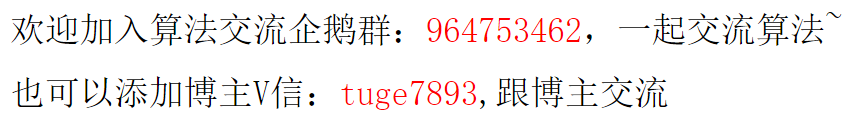# 1. 高斯函数与高斯滤波

G ( x ) = 1 2 π σ exp ⁡ ( − x 2 2 σ 2 ) G(x) = \frac{1}{\sqrt{2\pi}\sigma} \exp(-\frac{x^2}{2\sigma^2})

G ( x , y ) = 1 2 π σ 2 exp ⁡ ( − x 2 + y 2 2 σ 2 ) G(x, y) = \frac{1}{2\pi\sigma^2}\exp(-\frac{x^2+y^2}{2\sigma^2})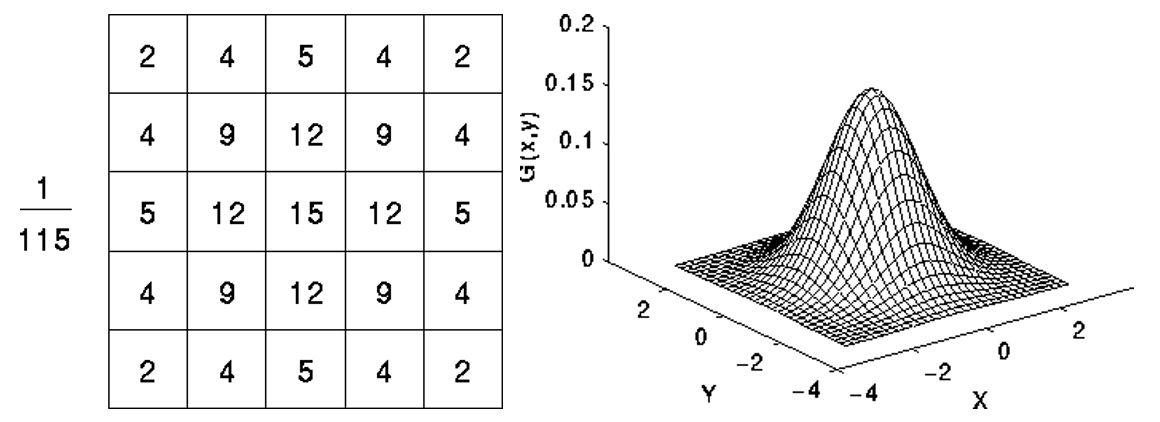# 2. 标准差

μ = 0 μ=0 时，唯一需要控制的参数就是标准差 σ σ ，多少合适呢？σ的确定十分依赖于问题背景，需要具体问题具体分析。但理解σ的作用，可以指导调整的方向。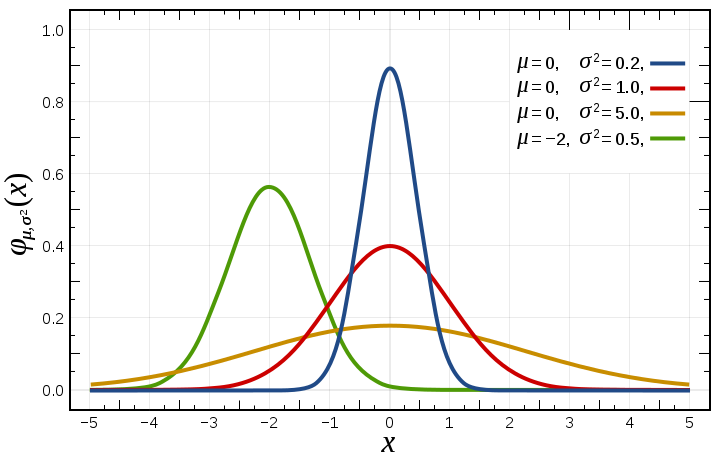σ x = 1 2 π σ w \sigma_x = \frac{1}{2\pi \sigma_w}

# 3. 窗口大小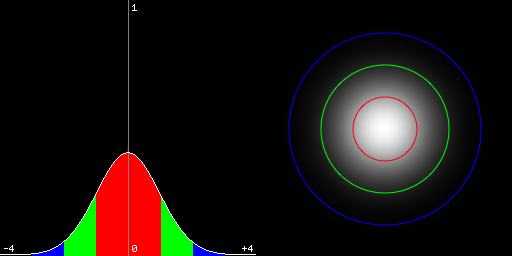# 4. OpenCV中标准差与窗口大小的换算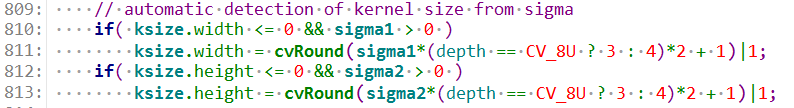σ = 0.3 × ( ( k s i z e − 1 ) × 0.5 − 1 ) + 0.8 \sigma = 0.3\times((ksize - 1)\times0.5 - 1) + 0.8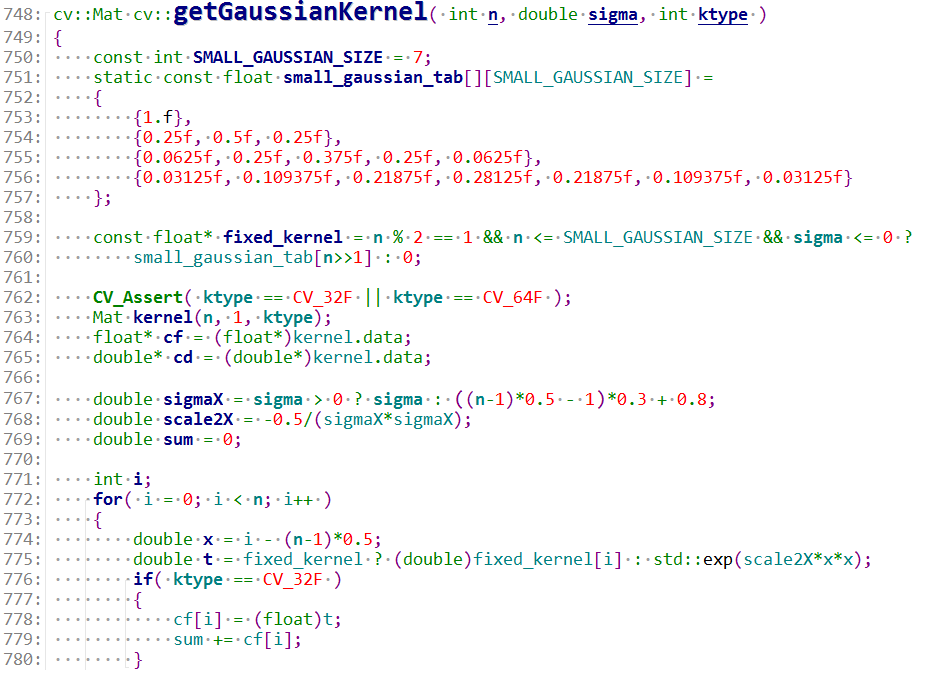G ( r , 0 ) G ( 0 , 0 ) = exp ⁡ ( − r 2 2 × ( 0.3 ( r − 1 ) + 0.8 ) 2 ) \frac{G(r, 0)}{G(0, 0)} = \exp(-\frac{r^2}{2 \times (0.3(r-1)+0.8)^2})

# 5. 最后暂无相关的数据...